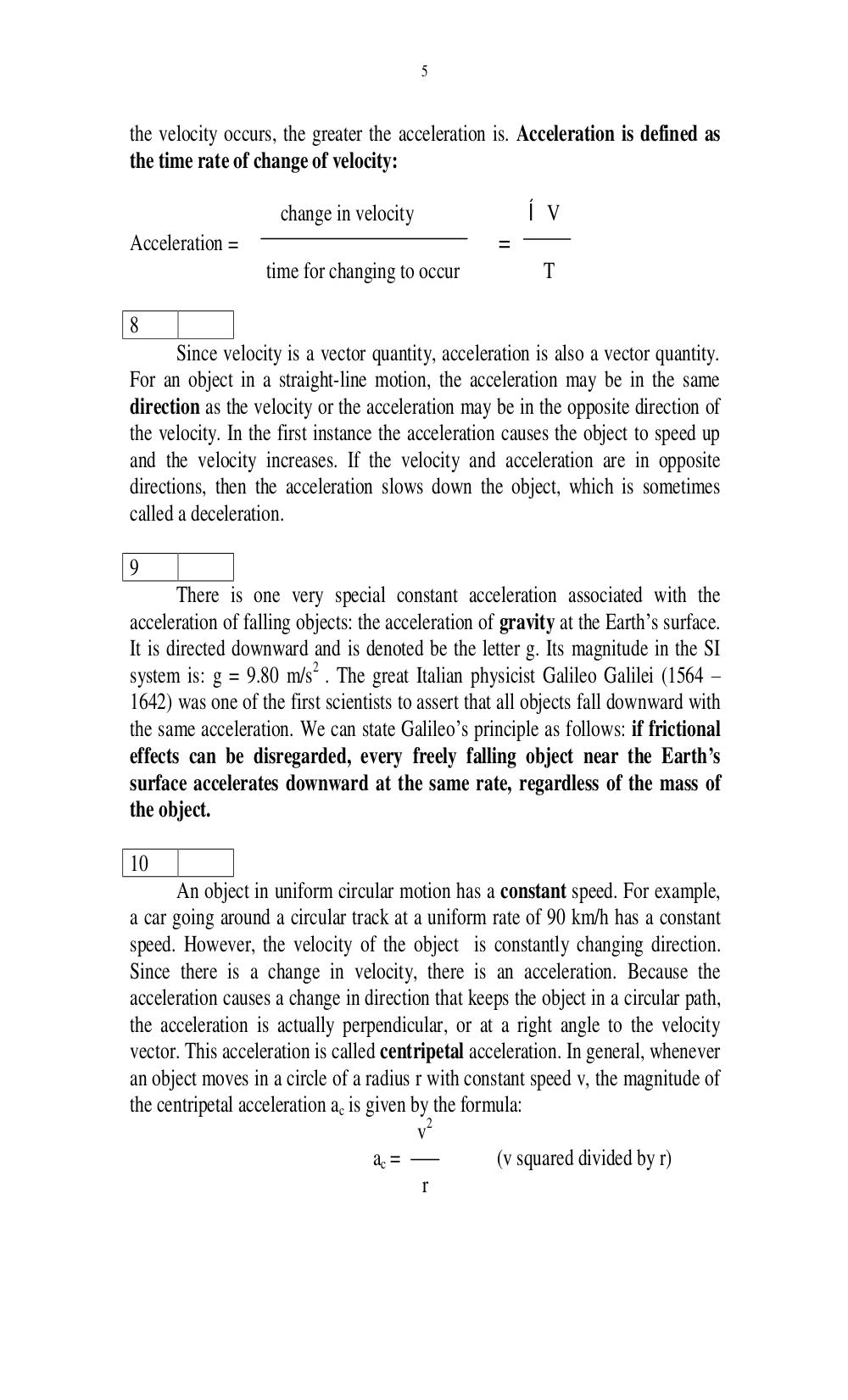# Английский язык. Ильичева Н.А - 5 стр.

Составители:

Рубрика:

• ## Иностранный язык5
the velocity occurs, the greater the acceleration is. Acceleration is defined as
the time rate of change of velocity:
change in velocity êV
Acceleration = =
time for changing to occur T
8
Since velocity is a vector quantity, acceleration is also a vector quantity.
For an object in a straight-line motion, the acceleration may be in the same
direction as the velocity or the acceleration may be in the opposite direction of
the velocity. In the first instance the acceleration causes the object to speed up
and the velocity increases. If the velocity and acceleration are in opposite
directions, then the acceleration slows down the object, which is sometimes
called a deceleration.
9
There is one very special constant acceleration associated with the
acceleration of falling objects: the acceleration of gravity at the Earths surface.
It is directed downward and is denoted be the letter g. Its magnitude in the SI
system is: g = 9.80 m/s
2
. The great Italian physicist Galileo Galilei (1564
1642) was one of the first scientists to assert that all objects fall downward with
the same acceleration. We can state Galileos principle as follows: if frictional
effects can be disregarded, every freely falling object near the Earths
surface accelerates downward at the same rate, regardless of the mass of
the object.
10
An object in uniform circular motion has a constant speed. For example,
a car going around a circular track at a uniform rate of 90 km/h has a constant
speed. However, the velocity of the object is constantly changing direction.
Since there is a change in velocity, there is an acceleration. Because the
acceleration causes a change in direction that keeps the object in a circular path,
the acceleration is actually perpendicular, or at a right angle to the velocity
vector. This acceleration is called centripetal acceleration. In general, whenever
an object moves in a circle of a radius r with constant speed v, the magnitude of
the centripetal acceleration a
c
is given by the formula:
v
2
a
c
= (v squared divided by r)
r5

the velocity occurs, the greater the acceleration is. Acceleration is defined as
the time rate of change of velocity:

change in velocity                 Í V
Acceleration =                                      =
time for changing to occur             T

8
Since velocity is a vector quantity, acceleration is also a vector quantity.
For an object in a straight-line motion, the acceleration may be in the same
direction as the velocity or the acceleration may be in the opposite direction of
the velocity. In the first instance the acceleration causes the object to speed up
and the velocity increases. If the velocity and acceleration are in opposite
directions, then the acceleration slows down the object, which is sometimes
called a deceleration.

9
There is one very special constant acceleration associated with the
acceleration of falling objects: the acceleration of gravity at the Earth’s surface.
It is directed downward and is denoted be the letter g. Its magnitude in the SI
system is: g = 9.80 m/s2 . The great Italian physicist Galileo Galilei (1564 –
1642) was one of the first scientists to assert that all objects fall downward with
the same acceleration. We can state Galileo’s principle as follows: if frictional
effects can be disregarded, every freely falling object near the Earth’s
surface accelerates downward at the same rate, regardless of the mass of
the object.

10
An object in uniform circular motion has a constant speed. For example,
a car going around a circular track at a uniform rate of 90 km/h has a constant
speed. However, the velocity of the object is constantly changing direction.
Since there is a change in velocity, there is an acceleration. Because the
acceleration causes a change in direction that keeps the object in a circular path,
the acceleration is actually perpendicular, or at a right angle to the velocity
vector. This acceleration is called centripetal acceleration. In general, whenever
an object moves in a circle of a radius r with constant speed v, the magnitude of
the centripetal acceleration ac is given by the formula:
v2
ac =            (v squared divided by r)
r